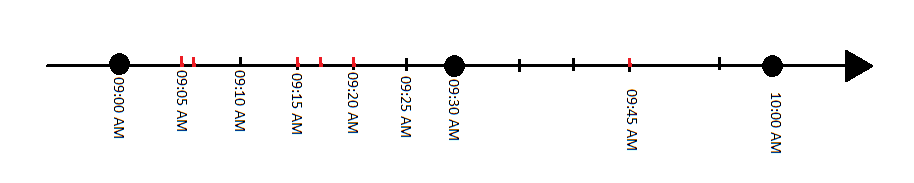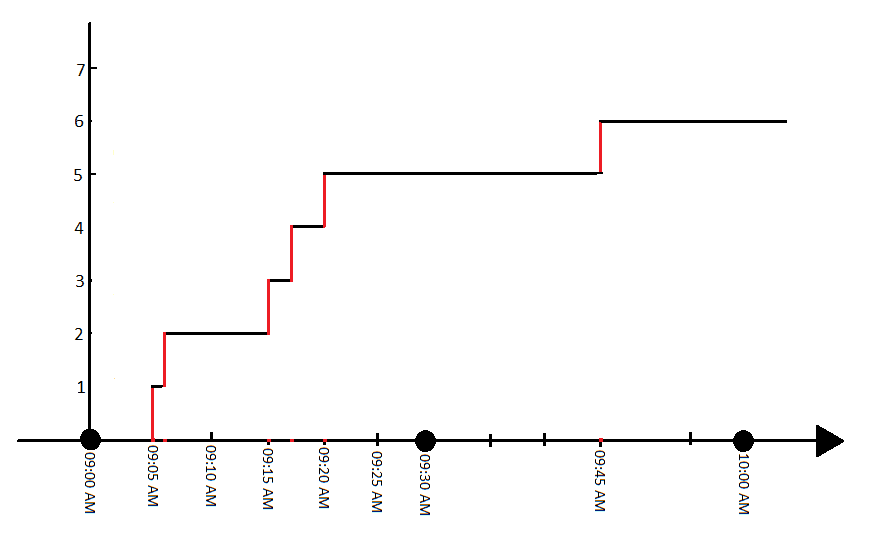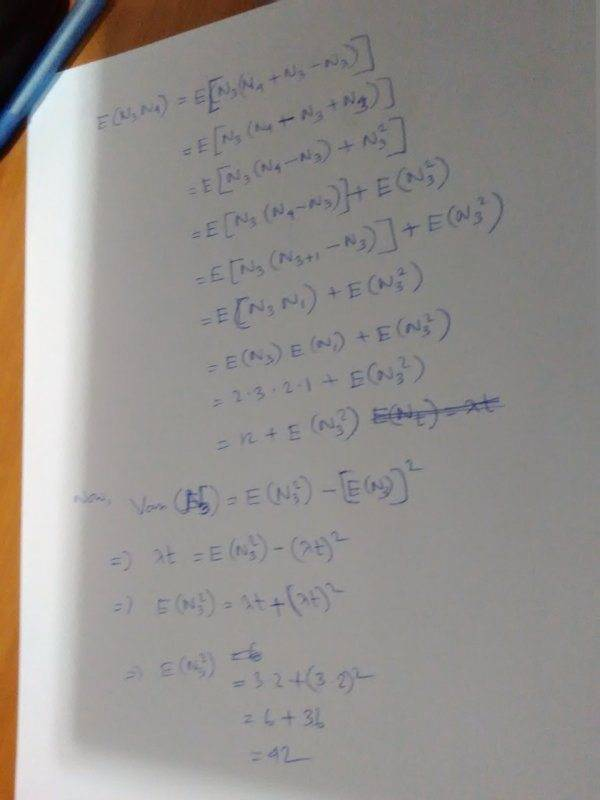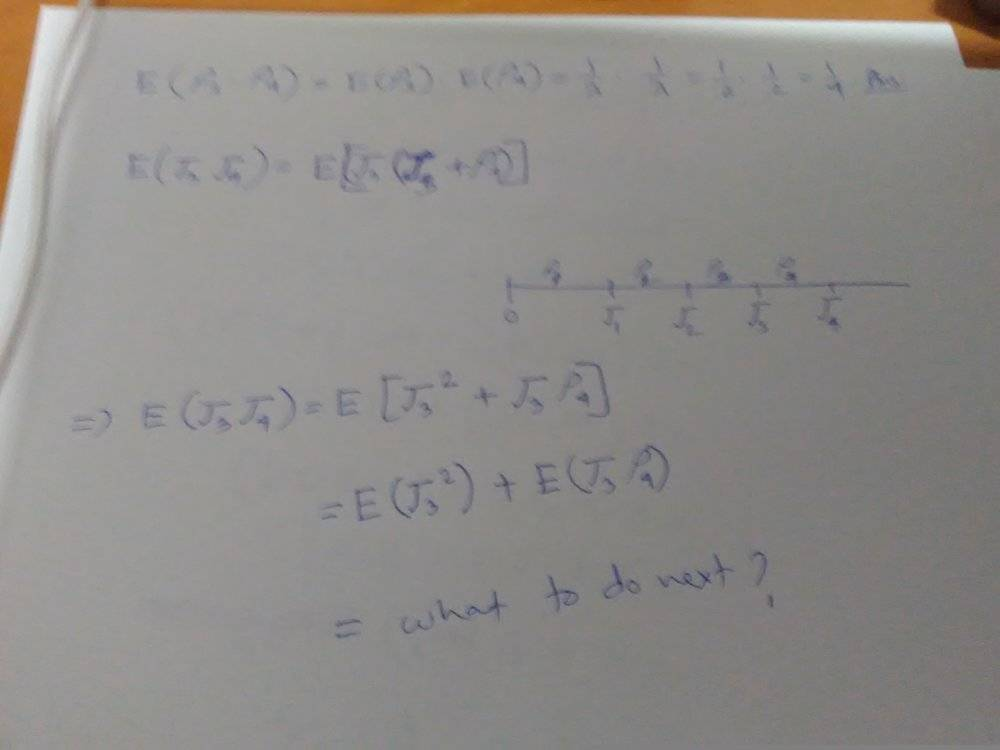# What is the difference between time, arrival-time, and inter-arrival-time is Poisson process?

• I
Gold Member
Let ##(N_t)_t## be a Poisson process with parameter ##λ = 2##. By ##τ_k## denote the time of the ##k##-th arrival ##(k = 1, 2, . . .)##, and by ##ρ_k=τ_k−τ_{k−1}## − the inter arrival time between the ##(k−1)## th and ##k##th arrival ##(k=1,2,...)##, with ##ρ_k=τ_k−τ_k−1##−the inter arrival time between the ##(k−1)## th and ##k## th arrival ##(k=1,2,...)##, with ##τ_0 = 0## (as in the construction of Poisson process).

Find the following:
(a) ##E(N_3N_4)##
(b) ##E(ρ_3ρ_4)##
(c) ##E(τ_3τ_4)##
.
Calls are received at a company call center according to a Poisson process at the rate of five calls per minute.
(a) Find the probability that no call occurs over a 30-second period.
(b) Find the probability that exactly four calls occur in the first minute, and six calls occur in the second minute.
(c) Find the probability that 25 calls are received in the first 5 minutes and six of those calls occur in the first minute.
The above are some of the typical problems related to Poisson Process. I need to understand the difference between time, inter-arrival time, and arrival time in this regard.

Say, we start our counting from 9:00 AM and count up to 10:00 AM.Image-1: arrival process.

1. 1st call comes at 09:05 AM
2. 2nd call comes at 09:06 AM
3. 3rd call comes at 09:15 AM
4. 4th call comes at 09:17 AM
5. 5th call comes at 09:20 AM
6. 6th call comes at 09:45 AMImage-2: Poisson counting process

If (Nt)t(Nt)t is the Poisson process, what would be the values of tt?

What would be the time, arrival time, and inter-arrival time in this example?

As far as I understand:

* 1 minute, 9 minutes, 2 minutes, 3 minutes, 25 minutes are inter-arrival-times ##ρ_k##.

Are [0,5] minutes, [0,6] minutes, [0,15] minutes, ... etc time or arrival time?

Gold Member
Let ##(N_t)_t## be a Poisson process with parameter ##λ = 2##. By ##τ_k## denote the time of the k-th arrival (k = 1, 2, . . .), and by ##ρ_k = τ_k −τ_{k−1}## - the interarrival time between the (k−1)th and kth arrival (k = 1, 2, . . .), with ##τ_0 = 0## (as in the construction of Poisson process).

Find the following:

(a) ##E(N_3N_4)##
(b) ##E(ρ_3ρ_4)##
(c) ##E(τ_3τ_4)##

I can understand (a), and (b). But, couldn't understand (c).

I collected the following from a friend:
(c)

##E(τ_3τ_4)##
##= E[τ_3(τ_3+ρ_4)]##
##= E(τ^2_3 + ρ_4)##
##= E(τ^2_3) + E(ρ_4)##
##= (k/\lambda)^2 + 1/ \lambda ##

First of all, is this a correct solution? If not, what is the correct one?

The processing of ##τ_3## doesn't look okay to me.

It seems to me that ##ρ_4## is introduced here because ##τ_3## and ##τ_4## are not independent. Why are not they independent?

Suppose, the 3rd item arrived at 9:05 AM and the 4th one arrived at 9:10 AM. If the process started at 9:00 AM, the 3rd one took 5 minutes and the 4th one took 10 minutes to arrive.

How are they not independent?

Gold Member
I again mention this site:

http://www.randomservices.org/random/poisson/Introduction.html

If you get stuck with Poisson processes, you may want to consider the exact same problem in this discrete time counterpart -- a Bernouli Process. It is fruitful to toggle between the two.

As for this
##E(N_3N_4)##

consider applying the Tower Property of conditional expectations, i.e.

##E\Big[N_3N_4\Big] = E\Big[E\big[ N_3N_4\big \vert N_3 \big]\Big]=E\Big[N_3 \cdot E\big[ N_4\big \vert N_3 \big]\Big]##

so what can you do with that? You've had 3 + posting now in the last day on memorylessness prior to this one. As a hint for the above: exploit memorylessness.

Gold Member
I again mention this site:

http://www.randomservices.org/random/poisson/Introduction.html

If you get stuck with Poisson processes, you may want to consider the exact same problem in this discrete time counterpart -- a Bernouli Process. It is fruitful to toggle between the two.

As for this
##E(N_3N_4)##

consider applying the Tower Property of conditional expectations, i.e.

##E\Big[N_3N_4\Big] = E\Big[E\big[ N_3N_4\big \vert N_3 \big]\Big]=E\Big[N_3 \cdot E\big[ N_4\big \vert N_3 \big]\Big]##

so what can you do with that? You've had 3 + posting now in the last day on memorylessness prior to this one. As a hint for the above: exploit memorylessness.

i have solved (a) and (b) myself.

Need some help with (c) only.

By the way, YouTube video of Neal Patwari was much more better than the link you supplied.

Gold Member
i have solved (a) and (b) myself.

Need some help with (c) only.
The thread is all over the place.

Please show your solution to (a). Then show how you tried a similar approach to (c) and failed. The exact same technique may be used against (c). This is why I think you are memorizing things and not mastering techniques or concepts.

Gold MemberGold Member